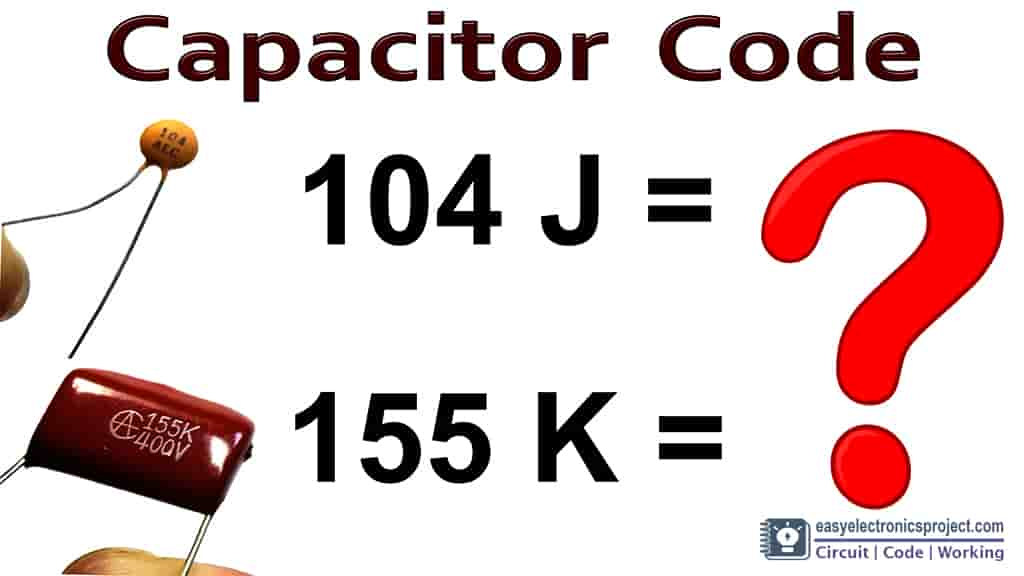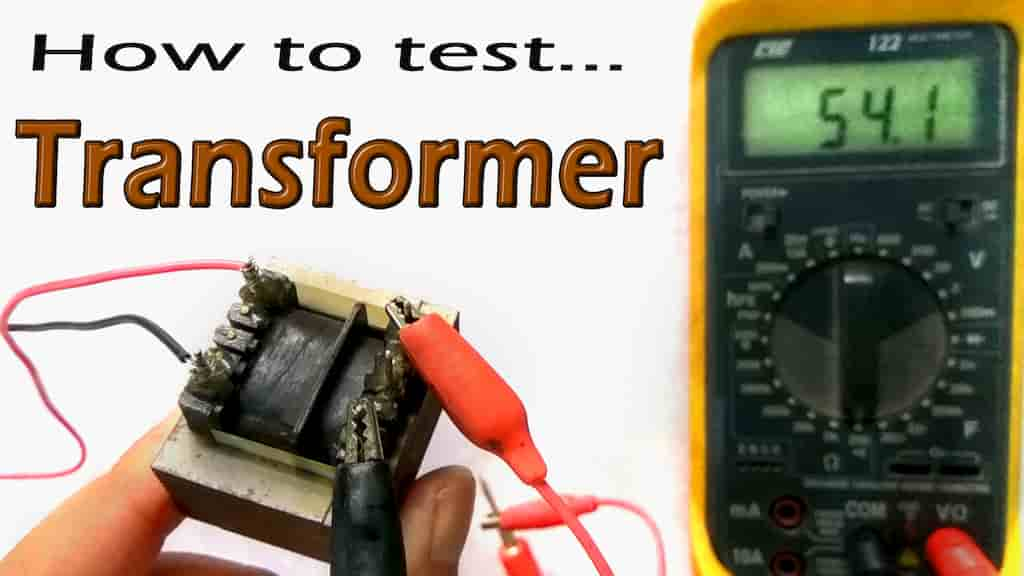## Tutorials:

Explore different tutorials on Basic Electronics  components like Resistor, Capacitor, Transistor, Diode, LED etc. Also explained how to use multimeter, how to test different types of capacitors, how to measure resistors accurately, how to identity PNP or NPN transistors, how to test MOSFET, how to test transformer using multimeter etc.

All tutorials explained in step by step and with practical examples.

## Capacitor Tutorials:#### Capacitor Code Calculation with chart

In this article, I have explained how to calculate the capacitance value from the 3-digit capacitor code. For the ceramic ...

## Transformer Tutorials:#### Transformer testing with Multimeter

In this tutorial, I have explained how to test step down transformer using the digital multimeter. This is not an ...